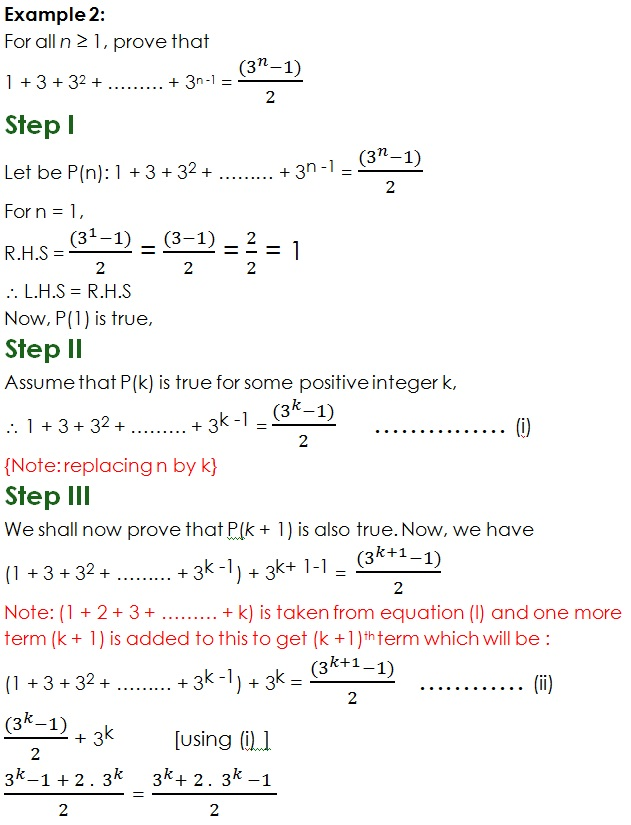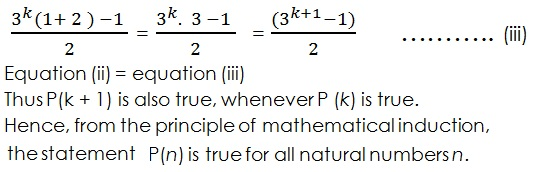Study Materials: CBSE Notes

Our CBSE Notes for Introduction Class 11 maths 4. Principle of Mathematical Induction - CBSE Notes - Toppers Study is the best material for English Medium students cbse board and other state boards students.

Notes ⇒ Class 11th ⇒ Mathematics ⇒ 4. Principle of Mathematical Induction

# Introduction Class 11 maths 4. Principle of Mathematical Induction - CBSE Notes - Toppers Study

Topper Study classes prepares CBSE Notes on practical base problems and comes out with the best result that helps the students and teachers as well as tutors and so many ecademic coaching classes that they need in practical life. Our CBSE Notes for Introduction Class 11 maths 4. Principle of Mathematical Induction - CBSE Notes - Toppers Study is the best material for English Medium students cbse board and other state boards students.

## Introduction Class 11 maths 4. Principle of Mathematical Induction - CBSE Notes - Toppers Study

CBSE board students who preparing for class 11 ncert solutions maths and Mathematics solved exercise chapter 4. Principle of Mathematical Induction available and this helps in upcoming exams 2023-2024.

### You can Find Mathematics solution Class 11 Chapter 4. Principle of Mathematical Induction

• All Chapter review quick revision notes for chapter 4. Principle of Mathematical Induction Class 11
• NCERT Solutions And Textual questions Answers Class 11 Mathematics
• Extra NCERT Book questions Answers Class 11 Mathematics
• Importatnt key points with additional Assignment and questions bank solved.

NCERT Solutions do not only help you to cover your syllabus but also will give to textual support in exams 2023-2024 to complete exercise maths class 11 chapter 4 in english medium. So revise and practice these all cbse study materials like class 11 maths chapter in english ncert book. Also ensure to repractice all syllabus within time or before board exams for ncert class 11 maths ex in english.

See all solutions for class 11 maths chapter 4 exercise 4 in english medium solved questions with answers.

### Introduction class 11 Mathematics Chapter 4. Principle of Mathematical Induction

• Introduction Class 11 Maths 4. Principle Of Mathematical Induction - CBSE Notes - Toppers Study
• Class 11 Ncert Solutions
• Solution Chapter 4. Principle Of Mathematical Induction Class 11
• Solutions Class 11
• Chapter 4. Principle Of Mathematical Induction Introduction Class 11

## Introduction Class 11 maths 4. Principle of Mathematical Induction - CBSE Notes - Toppers Study

4. Principle of Mathematical Induction

Mathematical induction is a technique for proving a statement, theorem, or a formula, that is asserted about every natural number.

The word induction means the generalisation from particular cases or facts.

Statement: A sentence which can be judged to be true or false is called statement.

Mathematical example:

(i) Eight is divisible by two.
(ii) Any number divisible by two is an even number,

therefore,

(iii) Eight is an even number.

Here statements (i) and (ii) are true, so

using (i) and (ii)

(iii) is also true

Deduction: Deduction is a process in which  a statement is given to be proven, often called a conjecture or a theorem in mathematics. deduction is the application of a general case to a particular case.

The Principle of Mathematical induction:

Concept:

(i) If a statement is true for one event then statement will be true for all given events.

This is the underlying principle of mathematical induction.

Steps:

Suppose there is a given statement P(n) involving the natural number n such that:

Step I:

First prove for P(1) where n =1 and n ∈ N;

When P(1) is true

then

Step II

Assume that P(k) is true for some positive integer k,

And replace n by k and make that a equation (I)

Step III

Then Add a extra term e.i (k +1) in equation I and replace n by (k + 1) from formula.

Then prove for (k + 1)##### Other Pages of this Chapter: 4. Principle of Mathematical Induction

Important Study materials for classes 06, 07, 08,09,10, 11 and 12. Like CBSE Notes, Notes for Science, Notes for maths, Notes for Social Science, Notes for Accountancy, Notes for Economics, Notes for political Science, Noes for History, Notes For Bussiness Study, Physical Educations, Sample Papers, Test Papers, Mock Test Papers, Support Materials and Books.Mathematics Class - 11th

NCERT Maths book for CBSE Students.

books

## Study Materials List:

##### Notes ⇒ Class 11th ⇒ Mathematics
1. Sets
2. Relations and Functions
3. Trigonometric Functions
4. Principle of Mathematical Induction
5. Complex Number and Quadratic Equations
6. Linear Inequalities
7. Permutations and Combinations
8. Binomial Theorem
9. Sequences and Series
10. Straight Lines
11. Conic Sections
12. Introduction to Three Dimensional Geometry
13. Limits and Derivatives
14. Mathematical Reasoning
15. Statistics
16. Probability

New Books